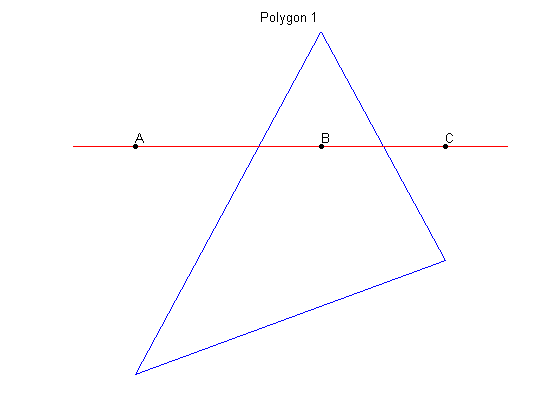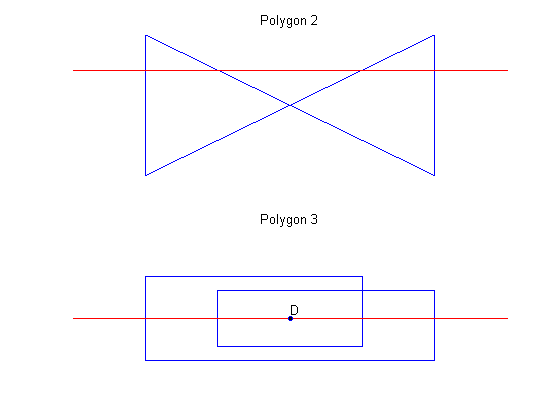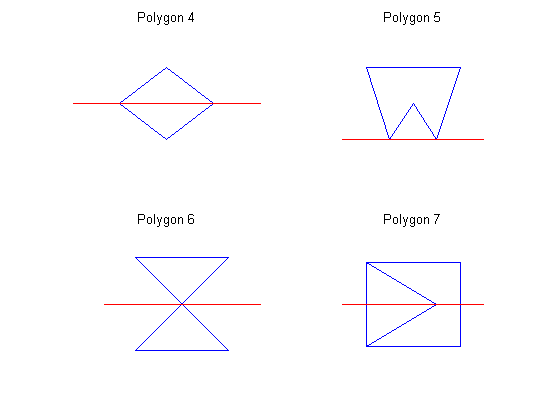# POLY2MASK and ROIPOLY – Part 2

I'm familiar with two basic ways to determine whether a point is inside a polygon. The first is to compute the winding number, which is the number of times that a closed curve encircles the point. This technique is used by the MATLAB function inpolygon.

When computing point-inside-polygon for all the points on a pixel grid, the crossing test is more commonly used. You follow a ray from one side of the image to the other, counting the number of times the ray crosses an edge of the polygon.

x1 = [30 80 60 30];
y1 = [30 50 90 30];
ray_x = [20 90];
ray_y = [70 70];
points_x = [30 60 80];
points_y = [70 70 70];
plot(x1,y1)
hold on
plot(ray_x,ray_y, 'r')
plot(points_x,points_y,'Marker','o','MarkerSize',4,'Color','k',...
'MarkerFaceColor','k','LineStyle','none')
text(30,70,'A','VerticalAlignment','bottom')
text(60,70,'B','VerticalAlignment','bottom')
text(80,70,'C','VerticalAlignment','bottom')
axis off
hold off
title('Polygon 1')At point A, the ray has not crossed a polygon edge; A is outside the polygon. At point B, the ray has crossed a polygon edge one time; B is inside. At point C, the ray has crossed a polygon edge twice; C is outside.

Polygons can get much more complex than a triangle, of course. Here are a couple of convex, nonsimple polygons.

subplot(2,1,1)
plot([30 70 70 30 30], [30 70 30 70 30])
hold on
plot([20 80],[60 60],'r')
hold off
axis off
title('Polygon 2')

subplot(2,1,2)
plot([30 70 70 40 40 60 60 30 30], ...
[30 30 55 55 35 35 60 60 30])
hold on
plot([20 80],[45 45],'r')
plot(50,45,'Marker','o','MarkerSize',4,'MarkerFaceColor','k')
text(50,45,'D','VerticalAlignment','bottom')
ylim([25 75])
hold off
axis off
title('Polygon 3')In polygon 2, the ray crosses into and then out of the polygon twice. Polygon 3 is even more interesting. Is point D inside or outside the polygon? The answer is a matter of convention. A commonly-used convention is the even-odd rule. A point is outside the polygon if there have been an even number of edge crossings. A point is inside there have been an odd number. By this rule, point D is outside the polygon.

The crossing test and the even-odd rule are easy to understand. The challenge is to avoid getting tripped up in the special cases. The troublesome cases include rays intersecting vertices, edge intersections, and coincident edges. Another case is when a ray is coincident with an edge. Here are some diagrams to illustrate. (Note that in polygon 7 there are two coincident edges on the left side of the polygon.)

subplot(2,2,1)
plot([20 30 40 30 20], [30 20 30 40 30]);
hold on
plot([10 50], [30 30], 'r')
hold off
axis off
title('Polygon 4'), ylim([10 50])

subplot(2,2,2)
plot([20 30 40 50 60 20], [30 10 20 10 30 30]);
hold on
plot([10 70], [10 10], 'r')
hold off
axis off
title('Polygon 5'), ylim([0 40])

subplot(2,2,3)
plot([20 50 20 50 20], [20 20 60 60 20]);
hold on
plot([10 60], [40 40], 'r')
hold off
axis off
title('Polygon 6'), ylim([10 70])

subplot(2,2,4)
plot([20 60 60 20 20 50 20 20], ...
[20 20 50 50 20 35 50 20]);
hold on
plot([10 70],[35 35],'r')
hold off
axis off
title('Polygon 7'), ylim([10 60])In my next post on this topic, I'll get into the specific algorithm used by the Image Processing Toolbox functions polymask and roipoly.

Here's Part 1 of this topic.

Published with MATLAB® 7.3

|

### Comments

To leave a comment, please click here to sign in to your MathWorks Account or create a new one.